Next: Dielectric Waveguides Up: Resonant Cavities and Waveguides Previous: Cylindrical Cavities

# Waveguides

Let us consider the transmission of electromagnetic waves along the axis of a waveguide, which is simply a long, axially symmetric, hollow conductor with open ends. In order to represent a wave propagating along the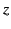-direction, we express the dependence of field quantities on the spatial coordinates and time in the form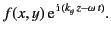(1354)

The guide propagation constant,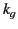, is just the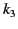of previous sections, except that it is no longer restricted by the boundary conditions to take discrete values. The general considerations of Section 10.5 still apply, so that we can treat TM and TE modes separately. The solutions forare identical to those for axially symmetric cavities already discussed. Althoughis not restricted in magnitude, we note that for every eigenvalue of the transverse wave equation,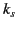, there is a lowest value of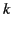, namely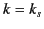(often designated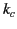for waveguides), for which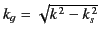is real. This corresponds to the cutoff frequency, below which waves are not transmitted by the mode in question, and the fields fall off exponentially with increasing. In fact, the waveguide dispersion relation for a particular mode can easily be shown to take the form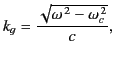(1355)

where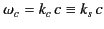(1356)

is the cutoff frequency. There is an absolute cutoff frequency associated with the mode of lowest frequency: that is, the mode with the lowest value of.

For real(i.e.,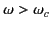), it is clear from Equation (1357) that the wave propagates along the guide at the phase velocity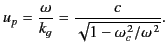(1357)

It is evident that this velocity is greater than that of electromagnetic waves in free space. The velocity is not constant, however, but depends on the frequency. The waveguide thus behaves as a dispersive medium. The group velocity of a wave pulse propagated along the guide is given by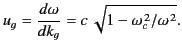(1358)

It can be seen that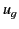is always smaller than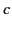, and also that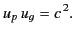(1359)

For a TM mode (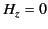), Equations (1342)-(1343) yield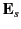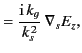(1360)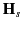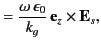(1361)

where use has been made of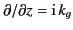. For TE modes (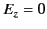), Equations (1344)-(1345) give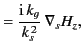(1362)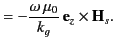(1363)

The time-averagecomponent of the Poynting vector,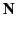, is given by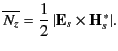(1364)

It follows that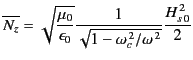(1365)

for TE modes, and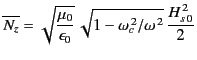(1366)

for TM modes. The subscript 0 denotes the peak value of a wave quantity.

For a given mode, waveguide losses can be estimated by integrating Equation (1319) over the wall of the guide. The energy flow of a propagating wave attenuates as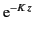, where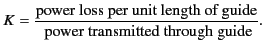(1367)

Thus,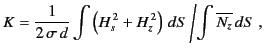(1368)

where the numerator is integrated over unit length of the wall, and the denominator is integrated over the transverse cross-section of the guide. It is customary to define the guide impedance,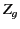, by writing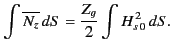(1369)

Here, both integrals are over the transverse cross-section of the guide. It follows from Equations (1366) and (1367) that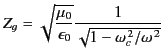(1370)

for TE modes, and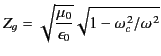(1371)

for TM modes. For both types of mode,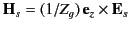.Next: Dielectric Waveguides Up: Resonant Cavities and Waveguides Previous: Cylindrical Cavities
Richard Fitzpatrick 2014-06-27